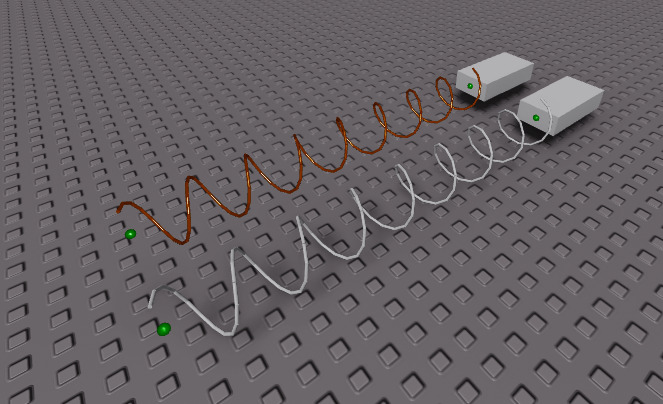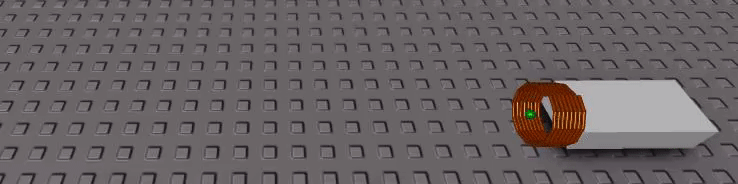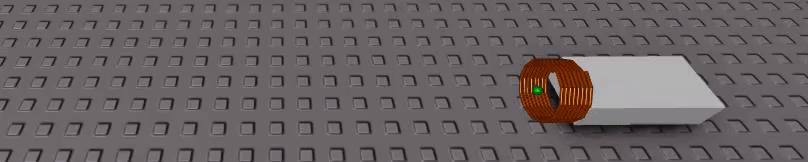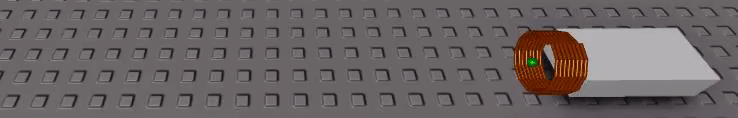# How can one write a function to visualize the visual shape of a SpringConstraint?

Hello,
I want to be able to calculate a function given number of coils, & length, to graph the visual model of a SpringConstraint when one updates those values.

These are some ways the visualization changes when I edit the number of coils:

And here’s how it looks when I change both length (from 0 to 15) & the number of coils (from 8 to 0), which is a unique type of motion:

I came along an article that mentioned different ways to calculate a harmonic spring-like function in 3D and 2D, but the functions don’t model the path correctly such as this one that I derived from one of the 3D functions:

``````local function getPointPosition(height, coils, t)
height = height or 0
coils = coils or 0
t = t or 0
--[[
spring3D[height_, n_, opts___] :=
ParametricPlot3D[
{Cos[n 2 Pi t], Sin[n 2 Pi t], height t},
{t, 0, 1},
Boxed -> False, Axes -> False,
opts]
]]
return Vector3.new(math.cos(coils * (2 * math.pi * t)), math.sin(coils * (2 * math.pi * t)), height * t)
end
``````

I’m wondering how I can write the function to model the spring to capture the end[any] point of the spring while it stretches & shrinks in coils as shown in the clip above?

1 Like

Do you mean finding the function of the spiral which the spring is made up of in 3D? Or do you mean the graph of how the spring oscillates and moves when a force is applied to it?

The function of the spiral in which the spring is made up of in 3D, as i’m trying to get the end point position (how it moves & wiggles) of the spiral in the video

A script along the lines of:

``````local fakeSpringPart = workspace.fakeSpringPart
local a0 = fakeSpringPart.Attachment0
local a1 = fakeSpringPart.Attachment1

local coils = 8
local thickness = 0.1

function spring(alpha)
local central = (a1.Position - a0.Position) * alpha
local angle = 2 * math.pi * alpha * coils

return central + offset
end

function make_part(cframe,size)
local p = Instance.new("Part")
p.Shape = Enum.PartType.Cylinder
p.Anchored = true
p.CanCollide = false
p.CFrame = cframe
p.Size = size
p.Parent = workspace
end

local last = spring(0)
for alpha = 1, coils > 0 and 12 * coils or 1, 1 do
local pos = spring(alpha/(coils > 0 and (12 * coils) or 1))

cframe = a0.WorldCFrame * CFrame.new((pos + last)/2, pos) * CFrame.Angles(0, math.pi/2, 0)
size = Vector3.new((pos - last).Magnitude, thickness, thickness)

last = pos

make_part(cframe, size)
end
``````

Should do the trickThe length is just calculated as the distance between the two attachments like it would be normally

Im not exactly sure this is what you mean but I can make adjustments if you need, just reply

1 Like

Oh I think I just realized what you mean
Do you think the code above will work fine for what you want?
Because I can simplify it a ton if you literally just want the endpoint as it extends and the number of coils decrease

Ill be gone for about an hour but I can write that code when I get back

1 Like

Your code block example to graph the SpringConstriant works really well, it models the coil exactly how roblox’s version looks especially when I’m editing the Coils and Length value indefinitely.

Indeed you have created for me exactly what I was looking for and took me longer to reply since I was experimenting with the code since, but I am realizing now that the end point (the tip of that wiggling end piece) is indeed the point I need

I was also meaning to ask how you derived the solution to the problem? I’m familiar with your use of sine and cosine to rotate along like a spring, but what was the thought process to evaluate your answer?

Here’s how accurate your graphing function is! [green = spring visualized by parts using your function, black = roblox’s SpringConstraint]

I used a slight variation of the normal spring function to get just the end point
This will technically work for any point along the spring too if you so desire

alpha = 1 (endpoint)alpha = 0.5 (midpoint)You can get even weirder cool effects by setting the alpha to changing values, this one changes alpha from 0 to 1 at the same rate that the length is changed
Pretty cool but also not the most practical thing in the worldHeres the code:

``````local real = workspace.real
local a0 = real.Attachment0
local a1 = real.Attachment1
local constraint = real.SpringConstraint

function spring_manual(alpha,coils) --very slightly modified spring() function to make it easier to edit coils real-time
local central = (a1.Position-a0.Position)*alpha
local angle = 2*math.pi*alpha*coils

return central+offset
end

for i = 0,1,0.01 do --just sample code that uses the above function as a proof of concept, not part of the math
constraint.Coils = (1 - i) * 8
a1.Position = a0.Position - Vector3.new(i * 15 + 1,0,0)
--note that the first argument is alpha in spring_manual()
--so you might do spring_manual(1, (1 - i) * 8) for endpoint
--or spring_manual(0.5, (1 - i) * 8) for midpoint
--or spring_manual(i, (1 - i) * 8) for the weird thing
workspace.marker.CFrame = a0.WorldCFrame * CFrame.new(spring_manual(1, (1 - i) * 8))

wait()
end
``````

As for the process of making it, I just started by inspecting the spring roblox made and saw that each coil was split into 12 segments when full
I then just made some code that consecutively made a spring thing using sin and cos split into 12 equally sized segments
You can just split the depth (or alpha value) into 12 segments per coil and you dont actually have to calculate the length of each segment because the relative change between each segment along the curve is the exact same
Beyond that its pretty straight forward, the rest of the details mostly just fell into place after making the basic system since roblox really doesnt use many fancy extra details (I even managed to “accidentally” recreate the height offset when coils are 0, but thats really just a result of the type of algorithm used)

But yeah hopefully that works fine for you

2 Likes